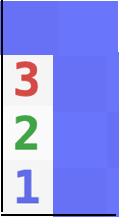# A Simple Minesweeper Puzzle

Suppose that you are playing the game Minesweeper.  On your first move, you click on the lower left corner square and reveal a 1.  Then you click on the square above the corner and reveal a 2.  Then you click on the square above that and reveal a 3.  What is the “safest” next move?In order to talk about the contents of the blue squares, we will label them A,B,C,D, and E.There are only three possible scenarios:

a) A, B, C, and E have mines,

b) A, C, and D have mines, or

c) B, C, and D have mines.

But, not all of these scenarios are equally likely.  Suppose there are a total of $m$ mines on the board and $s$ squares left excluding the eight that we are looking at. Then the total number of possible distributions of the mines for scenarios a, b, and c are:

• $n_a = {s\choose m-4},$
• $n_b= {s\choose m-3},$ and
• $n_c ={s\choose m-3}.$

These scenarios are not equally likely.  (Above we used choose notation.  ${n\choose m}= \frac{n!}{m! (n-m)!}$ where $n!=1\cdot2\cdot3\cdot\cdots\cdot n$.  For example 4!=24 and ${5\choose 2}=\frac{5!}{2!\ \cdot\ 3!} = \frac{120}{2 \cdot 6}= 10$.)  In fact,

\begin{aligned} r=\frac{n_b}{n_a}&=\frac{s\choose m-3}{s\choose m-4} \\&=\frac{\frac{s!}{(m-3)! (s-(m-3))!}}{\frac{s!}{(m-4)! (s-(m-4))!}}\\&=\frac{\frac{1}{(m-3)! (s-(m-3))!}}{\frac{1}{(m-4)! (s-(m-4))!}}\\&= \frac{(m-4)! (s-(m-4))!}{(m-3)! (s-(m-3))!}\\&= \frac{ (s-m+4)!}{(m-3) (s-m+3))!}\\&= \frac{ s-m+4}{m-3 }.\end{aligned}

In the beginning of the game $r\approx s/m-1\approx 4$, so scenarios b and c are about four times as likely as scenario a.  We can now estimate the probabilities of scenarios a, b, and c to be about

• “probability of scenario a” = $p_a \approx 1/9,$
• “probability of scenario b” = $p_b \approx 4/9, and$
• “probability of scenario c” = $p_c \approx 4/9.$

We can now conclude that the probability that square A has a mine is 5/9, that square B has a mine is 5/9, that square C has a mine is 100%, that square D has a mine is 8/9, and that square E has a mine is 1/9, so square E is the “safest” move.

Generally speaking, scenarios with more mines are less likely if less than half of the unknown squares have mines.

Another interesting way to think about it is that the 3 and 2 squares pulled the mines toward them making square E less likely to contain a mine.

You can approximate the probability of each scenario by just assuming that the squares are independent random variables (a false, but almost true assumption) each of which has probability $m/s$ of containing a mine.  Using that method gives the same results as the approximation above.

If you prefer an exact calculation, then use the formula

$$r=\frac{ s-m+4}{m-3 }$$

to get the exact ratio of $\frac{p_b}{p_a} = \frac{p_c}{p_a}=r$.

(PS:  Jennifer told me via email that you can play Minesweeper online at https://www.solitaireparadise.com/games_list/minesweeper.htm)